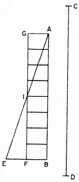A Source Book in Mathematics, 1200-1800

Contents:
Author: Galileo Galilei

Show Summary
Mathematics

4 GALILEI.

# Accelerated Motion

### THEOREM I, PROPOSITION I

The time in which any space is traversed by a body starting from rest and uniformly accelerated is equal to the time in which that same space would be traversed by the same body moving at a uniform speed whose value is the mean of the highest speed and the speed just before acceleration began.

Let us represent by the line AB [Fig. 1] the time in which the space CD is traversed by a body which starts from rest, at C and is uniformly accelerated; let the final and highest value of the speed gained during the interval AB be represented by the line EB drawn at right angles to AB; draw the line AE, then all lines drawn from equidistant points on AB and parallel to BE will representFig. 1

the increasing values of the speed, beginning with the instant A. Let the point F bisect the line EB; draw FG parallel to BA, and GA parallel to FB, thus forming a parallelogram AGFB which will be equal in area to the triangle AEB, since the side GF bisects the side AE at the point I; for if the parallel lines in the triangle AEB are extended to GI, then the sum of all the parallels contained in the quadrilateral is equal to the sum of those contained in the triangle AEB; for those in the triangle IEF are equal to those contained in the triangle GIA, while those included in the trapezium AIFB are common. Since each and every instant of time in the time interval AB has its corresponding point on the line AB, from which points parallels drawn in and limited by the triangle AEB represent the increasing values of the growing velocity, and since parallels contained within the rectangle represent the values of a speed which is not increasing, but constant, it appears, in like manner, that the momenta assumed by the moving body may also be represented, in the case of the accelerated motion, by the increasing parallels of the triangle AEB, and, in the case of the uniform motion, by the parallels of the rectangle GB. For what the momenta may lack in the first part of the accelerated motion (the deficiency of the momenta being represented by the parallels of the triangle AGI ) is made up by the momenta represented by the parallels of the triangle IEF.

Hence it is clear that equal spaces will be traversed in equal times by two bodies, one of which, starting from rest, moves with a uniform acceleration, while the momentum of the other, moving with uniform speed, is one-half its maximum momentum under accelerated motion. Q.E.D.

Contents:

### Related Resources

Galileo Galilei

Title: A Source Book in Mathematics, 1200-1800

Select an option:

## Email Options

Title: A Source Book in Mathematics, 1200-1800

Select an option: